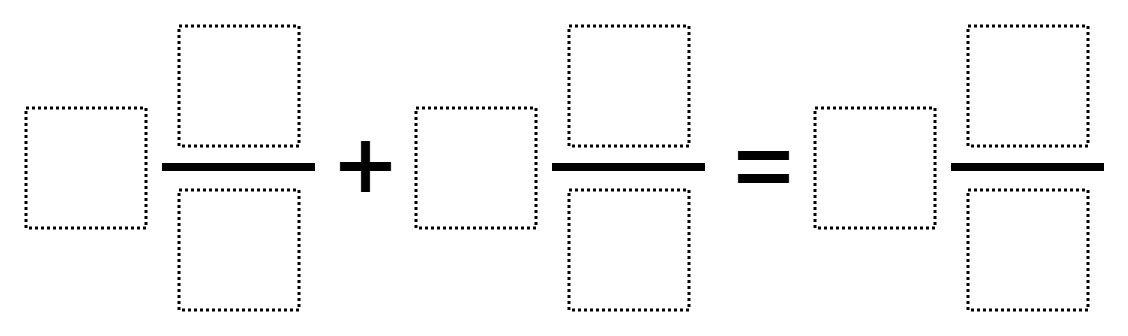Directions: Use the digits 1-9 each once to make a true statement.### Hint

What assumptions are we making about the fractions we are able to use? Do fractions have to be in lowest terms?

Number of Unique Solutions: 100
1: 1 3/2 + 7 6/8 = 9 5/4
2: 1 3/9 + 4 7/2 = 8 5/6
3: 1 4/2 + 5 7/3 = 9 8/6
4: 1 4/3 + 8 7/6 = 9 5/2
5: 1 4/6 + 7 3/9 = 5 8/2
6: 1 5/2 + 3 8/4 = 7 9/6
7: 1 5/2 + 4 8/3 = 9 7/6
8: 1 5/2 + 7 3/6 = 9 8/4
9: 1 5/2 + 7 4/8 = 9 6/3
10: 1 5/3 + 7 8/6 = 9 4/2
11: 1 5/6 + 4 9/2 = 8 7/3
12: 1 6/3 + 7 2/8 = 9 5/4
13: 1 6/8 + 2 9/3 = 5 7/4
14: 1 7/3 + 5 4/2 = 9 8/6
15: 1 7/6 + 8 4/3 = 9 5/2
16: 1 8/3 + 4 5/2 = 9 7/6
17: 1 8/6 + 7 5/3 = 9 4/2
18: 1 9/3 + 2 6/8 = 5 7/4
19: 1 9/3 + 5 4/8 = 6 7/2
20: 1 9/6 + 5 2/8 = 7 3/4
21: 1 9/6 + 5 3/2 = 7 8/4
22: 2 1/3 + 7 4/8 = 9 5/6
23: 2 1/6 + 5 3/9 = 7 4/8
24: 2 3/1 + 5 6/8 = 9 7/4
25: 2 3/9 + 5 1/6 = 7 4/8
26: 2 4/8 + 9 3/6 = 5 7/1
27: 2 4/8 + 9 3/6 = 7 5/1
28: 2 5/1 + 3 6/8 = 9 7/4
29: 2 6/8 + 3 5/1 = 9 7/4
30: 2 6/8 + 5 9/4 = 3 7/1
31: 2 6/9 + 8 4/3 = 7 5/1
32: 2 7/3 + 5 1/6 = 9 4/8
33: 2 9/4 + 5 6/8 = 7 3/1
34: 3 2/1 + 5 6/8 = 9 7/4
35: 3 2/8 + 5 1/4 = 7 9/6
36: 3 4/8 + 5 9/2 = 6 7/1
37: 3 5/2 + 1 8/4 = 7 9/6
38: 3 6/8 + 5 2/1 = 9 7/4
39: 3 9/2 + 5 4/8 = 6 7/1
40: 3 9/2 + 5 4/8 = 7 6/1
41: 4 2/6 + 7 8/3 = 9 5/1
42: 4 2/6 + 9 5/3 = 7 8/1
43: 4 6/2 + 5 9/3 = 7 8/1
44: 4 6/2 + 5 9/3 = 8 7/1
45: 4 8/3 + 7 2/6 = 5 9/1
46: 4 8/3 + 7 2/6 = 9 5/1
47: 4 9/3 + 5 6/2 = 7 8/1
48: 4 9/3 + 5 6/2 = 8 7/1
49: 5 1/2 + 3 6/8 = 7 9/4
50: 5 2/4 + 9 3/6 = 7 8/1
51: 5 2/4 + 9 3/6 = 8 7/1
52: 5 2/8 + 3 1/4 = 7 9/6
53: 5 3/1 + 2 6/8 = 9 7/4
54: 5 3/6 + 9 2/4 = 7 8/1
55: 5 3/6 + 9 2/4 = 8 7/1
56: 5 4/2 + 1 3/9 = 7 8/6
57: 5 4/6 + 7 1/3 = 9 8/2
58: 5 6/4 + 1 7/2 = 8 9/3
59: 5 6/4 + 8 3/2 = 7 9/1
60: 5 6/4 + 8 3/2 = 9 7/1
61: 5 6/8 + 2 9/4 = 3 7/1
62: 5 6/8 + 3 1/2 = 7 9/4
63: 5 7/2 + 1 6/4 = 8 9/3
64: 5 7/3 + 2 1/6 = 9 4/8
65: 5 9/2 + 3 4/8 = 7 6/1
66: 5 9/3 + 1 4/8 = 6 7/2
67: 5 9/4 + 2 6/8 = 7 3/1
68: 5 9/6 + 1 2/8 = 7 3/4
69: 5 9/6 + 1 3/2 = 7 8/4
70: 6 2/8 + 7 3/4 = 5 9/1
71: 6 4/8 + 1 5/2 = 7 9/3
72: 6 5/2 + 1 4/8 = 7 9/3
73: 7 2/3 + 1 5/6 = 9 4/8
74: 7 2/4 + 1 3/9 = 8 5/6
75: 7 2/8 + 1 5/4 = 9 3/6
76: 7 2/8 + 6 3/4 = 5 9/1
77: 7 2/8 + 6 3/4 = 9 5/1
78: 7 3/2 + 1 6/8 = 9 5/4
79: 7 3/4 + 1 6/8 = 5 9/2
80: 7 3/4 + 6 2/8 = 9 5/1
81: 7 4/6 + 1 3/9 = 5 8/2
82: 7 4/6 + 5 1/3 = 9 8/2
83: 7 5/2 + 1 3/6 = 9 8/4
84: 7 5/2 + 1 4/8 = 9 6/3
85: 7 5/4 + 1 2/8 = 9 3/6
86: 7 5/6 + 1 2/3 = 9 4/8
87: 7 6/3 + 1 2/8 = 9 5/4
88: 7 6/8 + 1 3/4 = 5 9/2
89: 7 8/3 + 4 2/6 = 5 9/1
90: 8 4/3 + 2 6/9 = 5 7/1
91: 8 6/4 + 5 3/2 = 7 9/1
92: 8 6/4 + 5 3/2 = 9 7/1
93: 8 6/9 + 2 4/3 = 5 7/1
94: 8 6/9 + 2 4/3 = 7 5/1
95: 8 7/6 + 1 2/4 = 9 5/3
96: 9 2/6 + 4 5/3 = 7 8/1
97: 9 2/6 + 4 5/3 = 8 7/1
98: 9 4/8 + 2 3/6 = 5 7/1
99: 9 4/8 + 2 3/6 = 7 5/1
100: 9 5/3 + 4 2/6 = 8 7/1

Source: Ellen Metzger

## Multiplying Fractions 4

Directions: Using the digits 1 to 9 at most once each time, fill the boxes …

### One comment

1.Solution found by fifth grade students: 1-5/4 + 7-2/8 = 9-3/6